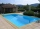Volume - 9th grade (14y) - examples

1. Three tributariesIt is possible to fill the pool with three tributaries. The first would take 12 hours, the second 15 hours, and the third 20 hours. The day before the summer season began, the manager opened all three tributaries simultaneously. How long did it take to fil
2. Lateral surface areaThe ratio of the area of the base of the rotary cone to its lateral surface area is 3: 5. Calculate the surface and volume of the cone, if its height v = 4 cm.The radius of the sphere we reduce by 1/3 of the original radius. How much percent does the volume and surface of the sphere change?
4. Sphere floatingWill float a hollow iron ball with an outer diameter d1 = 20cm and an inside diameter d2 = 19cm in the water? The iron density is 7.8 g/cm 3. (Instructions: Calculate the average sphere density and compare with the density of the water. )
5. Prism 4 sidesThe prism has a square base with a side length of 3 cm. The diagonal of the sidewall of the prism/BG/is 5 cm. Calculate the surface of this prism in cm square and the volume in liters
6. The diagram 2The diagram shows a cone with slant height 10.5cm. If the curved surface area of the cone is 115.5 cm2. Calculate correct to 3 significant figures: *Base Radius *Height *Volume of the cone
7. Pyramid heightFind the volume of a regular triangular pyramid with edge length a = 12cm and pyramid height h = 20cm.
8. PoolIf water flows into the pool by two inlets, fill the whole for 18 hours. The first inlet filled pool 6 hour longer than second. How long pool take to fill with two inlets separately?
9. TV transmitterThe volume of water in the rectangular swimming pool is 6998.4 hectoliters. The promotional leaflet states that if we wanted all the pool water to flow into a regular quadrangle with a base edge equal to the average depth of the pool, the prism would have.
10. Cube in a sphereThe cube is inscribed in a sphere with volume 6116 cm3. Determine the length of the edges of a cube.
11. Axial sectionAxial section of the cone is an equilateral triangle with area 208 dm2. Calculate the volume of the cone.
12. CuboidCuboid with edge a=16 cm and body diagonal u=45 cm has volume V=11840 cm3. Calculate the length of the other edges.
13. PoolThe swimming pool is 10 m wide and 8 m long and 153 cm deep. How many hectoliters of water is in it, if the water is 30 cm below its upper edge?
14. CubesOne cube is inscribed sphere and the other one described. Calculate difference of volumes of cubes, if the difference of surfaces in 257 mm2.
15. Transforming cuboidCuboid with dimensions 8 cm, 13, and 16 cm is converted into a cube with the same volume. What is its edge length?
16. SphereThe surface of the sphere is 2820 cm2, and weight is 71 kg. What is its density?
17. PipesWater pipe has a cross-section 1087 cm2. An hour has passed 960 m3 of water. How much water flows through the pipe with cross-section 300 cm2 per 9 hours if water flow same speed?
18. CylindersArea of the side of two cylinders is same rectangle of 50 cm × 11 cm. Which cylinder has a larger volume and by how much?
19. TanksFire tank has cuboid shape with a rectangular floor measuring 13.7 m × 9.8 m. Water depth is 2.4 m. Water was pumped from the tank into barrels with a capacity of 2.7 hl. How many barrels were used, if the water level in the tank fallen 5 cm? Wr
20. Cuboid diagonalCalculate the volume and surface area of the cuboid ABCDEFGH, which sides abc has dimensions in the ratio of 9:3:8 and if you know that the wall diagonal AC is 86 cm and angle between AC and the body diagonal AG is 25 degrees.

Do you have an interesting mathematical example that you can't solve it? Enter it, and we can try to solve it.

To this e-mail address, we will reply solution; solved examples are also published here. Please enter e-mail correctly and check whether you don't have a full mailbox.